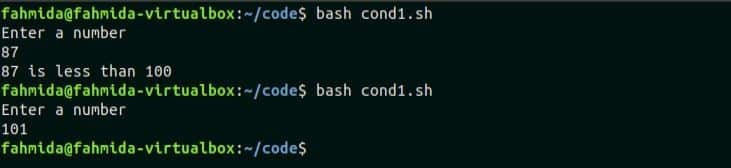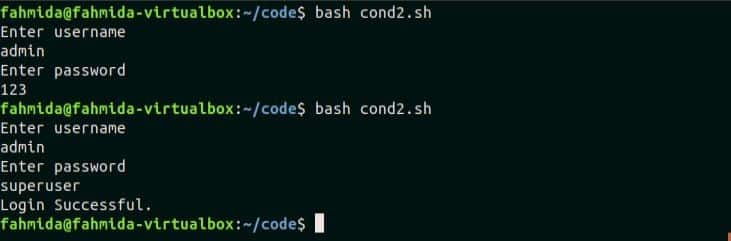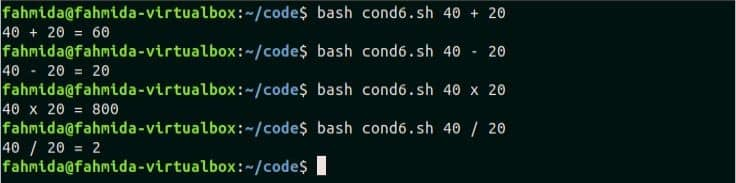BASH Programming

# Bash conditional statement

The conditional statement is used in any programming language to do any decision-making tasks. This statement is also used in bash to perform automated tasks like another programming language, just the syntax is a little bit different in bash. Two types of conditional statements can be used in bash. These are, ‘If’ and ‘case’ statements. ‘If’ statements can be used to check the conditions in multiple ways. Many variations of ‘if’ statements are described in this tutorial. ‘case’ statement is used as an alternative to ‘if’ statement. But, some specific conditions can be checked only by using ‘case’ statement and it doesn’t support various conditions like ‘if’ statement. So, any task done by ‘case’ statement can be done easily by ‘if’ statement, but ‘case’ statement can’t be used for all types of the task done by ‘if’ statement. This tutorial will help the readers to learn the uses of conditional statements in the bash script by using various examples.

### Types of conditional statements

The following types of conditional statements can be used in bash.

1. if statement
2. if else statement
3. if elif statement
4. Nested if statement
5. case statement

Each type of statements is explained in this tutorial with an example.

### Conditional operators

Many conditional operators can be used in ‘if’ statement to do any conditional task. Some mostly used conditional operators are mentioned below.

 Operator Description -eq Returns true if two numbers are equivalent -lt Returns true if a number is less than another number -gt Returns true if a number is greater than another number == Returns true if two strings are equivalent != Returns true if two strings are not equivalent ! Returns true if the expression is false -d Check the existence of a directory -e Check the existence of a file -r Check the existence of a file and read permission -w Check the existence of a file and write permission -x Check the existence of a file and execute permission

### Use of simple if statement

Syntax:

if [ condition ] ; then

Command(s)

fi

#### Example-1: if statement with the single condition

This example shows the single conditional use of if statement. Create a file named ‘cond1.sh’ and add the following script. This script will take a numeric value as input and check the value is less than 100 or not by using if condition. If the condition is true then it will print a message in the terminal.

cond1.sh

#!/bin/bash
echo "Enter a number"
if [ \$n -lt 100 ]; then
printf "\$n is less than 100\n"
fi

Output:

Run the script.

\$ bash cond1.sh

Here, 87 is taken as input which is less than 100. So, the output is “87 is less than 100”. No output is printed for the input greater than 100.#### Example-2: if statement with multiple conditions

How you can apply two conditions with logical AND in ‘if’ statement is shown in this example. Create a file named ‘cond2.sh’ and add the following script. Here, username and password will be taken from the user. Next, ‘if’ statement is used to check the username is ‘admin’ and the password is ‘superuser‘. If both values match then ‘if’ statement will return true and print the message “Login Successful”.

cond2.sh

#!/bin/bash
if [[ "\$un" == "admin" && "\$pw" = "superuser" ]]; then
fi

Output:

Run the script.

\$ bash cond2.sh

The script will print no output for invalid input and print the success message for valid input.### Use of if-else statement

Syntax:

if [ condition ]; then
Command(s)
else
Command(s)
fi

#### Example-3: if-else statement with multiple conditions

To execute one statement for true condition and another statement for the false condition, if-else statement is used in this example. Create a file named ‘cond3.sh’ and add the following script. Here, \$name variable is used to take input from the user and the value of \$name will be compared with two values, ‘Neha’ and ‘Nil’. If \$name matches with any of these values then if condition will return true and the statement of ‘if’ part will be executed. If \$name does not match with any of the values then if the condition will return false and the statement of ‘else’ part will be executed.

cond3.sh

#!/bin/bash
if [[ \$name == "Neha" || \$name == 'Nil' ]]; then
echo "You have won the prize"
else
echo "Try for the next time"
fi

Output:

Run the script.

\$ bash cond3.sh

The output is, “You have won the prize” for valid input and “Try for the next time” for the invalid input.### Use of if-elif-else statement

Syntax:

if [ condition ]; then
Command(s)
elif [ condition ]; then
Command(s)
…..
else
Command(s)
fi

#### Example-4: if-elif-else statement to check different conditions

Multiple conditions with multiple if statements are declared in this example to print grade based on input mark. Create a file named ‘cond4.sh’ and add the following script. After taking the value of \$mark, the first `if` statement will test the value is greater than or equal to 90. If it returns true then it will print “Grade – A+” otherwise it will go for the second condition. The second `if` will test the value is less than 90 and greater than or equal to 80. if it returns true then it will print “Grade – A” otherwise it will go for the third condition. If the third condition is true then it will print “Grade – B+” otherwise go for the fourth condition. If the fourth condition is true then it will print “Grade – C+” and if it returns false then the statement of else part will be executed that will print, “Grade – F”.

cond4.sh

#!/bin/bash
echo "Enter the mark"
if (( \$mark >= 90 )); then
elif (( \$mark < 90 && \$mark >= 80 )); then
elif (( \$mark < 80 && \$mark >= 70 )); then
elif (( \$mark < 70 && \$mark >= 60 )); then
else
fi

Output:

Run the script.

\$ bash cond4.sh

The script is tested by three mark values. These are 95, 79 and 50. According to the conditions used in the script,  the following output is printed.### Use of nested if

Syntax:

if [ condition ]; then
Commands
if [ condition ]; then
Commands
fi
fi

#### Example-5: Calculate bonus based on sales amount and duration

This example shows the use of nested if statement that will calculate bonus based on sales amount and time duration. Create a file named ‘cond5.sh’ and add the following code. The values of \$amount and \$duration are taken as input. Next, the first ‘if’ statement will check \$amount is greater than or equal to 10000 or not. If it returns true then it will check the condition of nested ‘if’statement. the value of \$duration is checked by the internal ‘if’ statement. If \$duration is less than or equal to 7 then “You will get 20% bolus” message will be stored otherwise the message “You will get 15% bonus” will be stored in the \$output variable. If the first ‘if’ condition returns false then the statements of else part will be executed. In the second nested ‘if’ condition, “You will get 10% bonus” message will print for returning a true value and “You will get 5% bonus” message will print for returning a false value.

cond5.sh

#!/bin/bash
echo "Enter the sales amount"
echo "Enter the time duration"

if (( \$amount >= 10000 )); then
if (( \$duration <= 7 )); then
output="You will get 20% bonus"
else
output="You will get 15% bonus"
fi
else
if (( \$duration <= 10 )); then
output="You will get 10% bonus"
else
output="You will get 5% bonus"
fi
fi
echo "\$output"

Output:

Run the script.

\$ bash cond5.sh

The script is tested by 12000 as amount and 5  as duration value first. According to the ‘if’ condition, the message, “You will get 20% bonus is printed. Next, the script is tested by 9000 as the amount and 12 as duration values and the message “You will get 5% bonus” is printed.### Use of case statement

Syntax:

case  in
pattern 1) commands;;
pattern n) commands;;
esac

#### Example-6: ‘case’ statement with a single value

‘case’ statement can be used as an alternative to ‘if’ statement. Create a file named ‘cond6.sh’ and add the following code to do some simple arithmetic operations. This script will read three values from the command line and store in the variables, \$N1, \$N2 and \$op. Here, \$N1 and \$N2 are used to store two numeric values and \$op is used to store any arithmetic operator or symbol. ‘case’ statement is used to check for four symbols to do any arithmetic operation. When \$op is ‘+’ then it will add \$N1 and \$N2 and store the result in \$Result. In the same way, ‘-‘ and ‘/’ symbols are used to do the subtraction and division operation. ‘x’ symbol is used here to do the multiplication operation. For any other value of \$op, it will print a message, “Wrong number of arguments”.

cond6.sh

#!/bin/bash
N1=\$1
op=\$2
N2=\$3
case \$op in
'+')
((Result=\$N1+\$N2)) ;;
'-')
((Result=\$N1-\$N2)) ;;
'x')
((Result=\$N1*\$N2)) ;;
'/')
((Result=\$N1/\$N2)) ;;
*)
echo "Wrong numbers of arguments"
exit 0 ;;
esac
echo "\$N1 \$op \$N2 = \$Result"

Output:

Run the script with three command line arguments. The script is executed for four times by using four operators, ‘+’, ’-’, ‘x’ and ‘/’.

\$ bash cond6.sh 40 + 20
\$ bash cond6.sh 40 - 20
\$ bash cond6.sh 40 x 20
\$ bash cond6.sh 40 / 20

The following output will appear after running the script.### Example-7: ‘case’ statement with a range of values

‘case’ statement can’t define multiple conditions with the logical operator like ‘if’ statement. But using the pipe (‘|’), multiple conditions can be assigned in the ‘case’ statement.  This example shows the grade value based on marks like Example-4 but using ‘case’ statement instead of ‘if’. \$name and \$mark values are given by command line arguments. The first condition is defined by ‘9[0-9]|100’ for printing “Grade – A+”. This means if the \$mark value is within 90-99 or 100 then the condition will be true. The second condition is ‘8[0-9]’ for printing “Grade – A” and this will match \$mark with any value from the range, 80-89.  The third and fourth conditions work like the second condition. The fifth condition is ‘0|1[0-9]|2[0-9]|3[0-9]|4[0-9]|5[0-9]’ for printing ‘Grade – F’ and it will match \$mark with 0 or any number in the ranges 0-9, 10-19, 20-29, 30-39, 40-49 and 50-59.

cond7.sh

#!/bin/bash
#   Print grade based on the mark
name=\$1
mark=\$2
case \$mark in
9[0-9]|100)
8[0-9])
7[0-9])
6[0-9])
0|[0-9]|1[0-9]|2[0-9]|3[0-9]|4[0-9]|5[0-9])
*)
echo "Invalid mark"
exit 0 ;;
esac

Output:

Run the script with two command line arguments. The script is run for four times with different argument values.

\$ bash cond7.sh Lily 92
\$ bash cond7.sh Nahar 78
\$ bash cond7.sh John 500
\$ bash cond7.sh John aa#### Conclusion:

Multiple uses of condition statements are tried to explain in this tutorial by using appropriate examples. Hope, the reader will be able to use conditional statements in bash script efficiently after practicing the above examples properly.